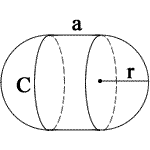## Solid Volume calculator

Find volume for:
 a = r = Units: Volume = 0a = side length
» Show Work

### Circular Cone Volume & Surface Area

1. Volume = (1/3)πr2h

### Capsule Volume

1. Volume = πr2((4/3)r + a)

### Circular Cylinder Volume

1. Volume = πr2h

1. Volume = a3

### Conical Frustum Volume

1. Volume = (1/3)πh (r12 + r22 + (r1 * r2))

### Pyramid Volume

1. Volume = (1/3)a2h

### Hemisphere Volume

1. Volume = (2/3)πr3

### Spherical Cap Volume

1. Volume = (1/3)πh2(3R - h)

### Rectangular Prism Volume

1. Volume = lwh

### Sphere Volume

1. Volume = (4/3)πr3

For example, To capsule, when a = 2, r = 1

1. To calculate the surface area of a capsule, use the following formula: 2πr(2r + a). When you substitute r and a for their values, you get 2π1(2 * 1 + 2), which equals 25.13274 m2.

For example, To hemisphere, when r = 2

1. To calculate the surface area of a capsule, use the following formula: 2πr(2r + a). When you substitute r and a for their values, you get 2π1(2 * 1 + 2), which equals 25.13274 m2.

Thinkcalculator.com provides you helpful and handy calculator resources.